# Conservative Validity

The main article on conformal prediction deduces the asymptotic conservative validity of conformal predictors from the corresponding result for smoothed conformal predictors. The following theorem asserts the conservative validity (in a non-asymptotic sense) of conformal predictors.

Theorem All conformal predictors are conservatively valid, i.e. for any exchangeable probability distribution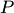on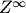there exists a probability space with two families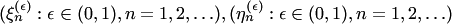of {0, 1}- valued random variables such that:

• for a fixed,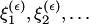is a sequence of independent Bernoulli random variables with the parameter;
• for all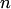and,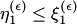;
• the joint distribution of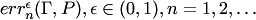coincides with the joint distribution of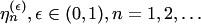, where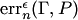is the random variable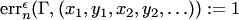if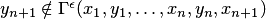;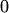otherwise.

Of course, this theorem also implies the asymptotic conservative validity of conformal predictors.

Corollary All conformal predictors are asymptotically conservative, i.e., for any exchangeable probability distributiononand any significance level,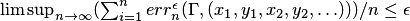with probability one.